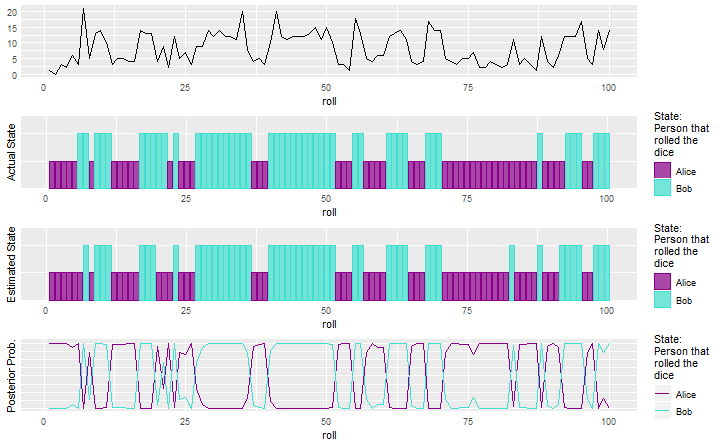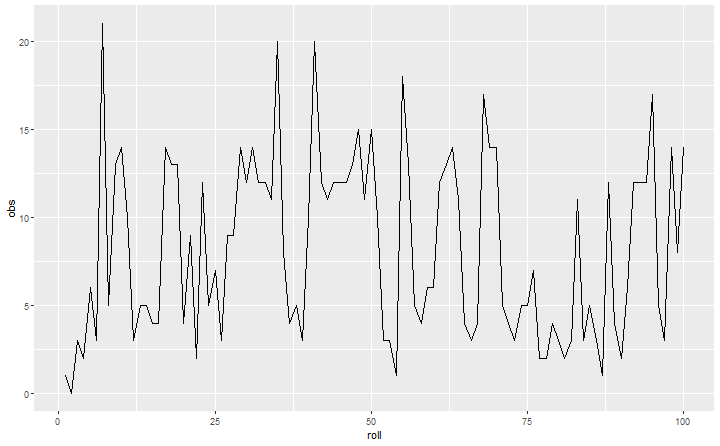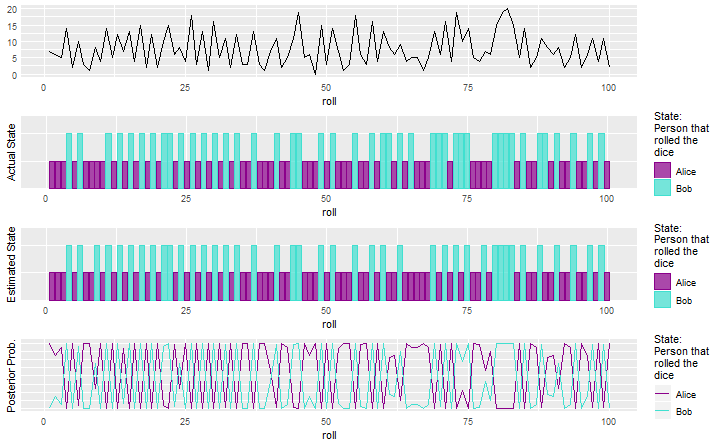Want to share your content on R-bloggers? click here if you have a blog, or here if you don't.Recently I developed a solution using a Hidden Markov Model and was quickly asked to explain myself. What are they and why do they work so well? I can answer the first part, the second we just have to take for granted.

HMM's are for modelling sequences of data whether they are derived from continuous or discrete probability distributions. They are related to state space and Gaussian mixture models in the sense they aim to estimate the state which gave rise to the observation. The states are unknown or 'hidden' and HMM's attempt to estimate the states similar to an unsupervised clustering procedure.

### The example

Before getting into the basic theory behind HMM's, here's a (silly) toy example which will help to understand the core concepts. There are 2 dice and a jar of jelly beans. Bob rolls the dice, if the total is greater than 4 he takes a handful of jelly beans and rolls again. If the total is equal to 2 he takes a handful jelly beans then hands the dice to Alice. It's now Alice's turn to roll the dice. If she rolls greater than 4 she takes a handful of jelly beans however she isn't a fan of any other colour than the black ones (a polarizing opinion) so puts the others back, therefore we would expect Bob to take more than Alice. They do this until the jar is empty.

Now assume Alice and Bob are in a different room and we can't see who is rolling the dice. Instead we only know how many jelly beans were taken after the roll. We don't know the colour, simply the final number of jelly beans that were removed from the jar on that turn. How could we know who rolled the dice? HMM's.

In this example the state is the person who rolled the dice, Alice or Bob. The observation is how many jelly beans were removed on that turn. The roll of the dice and the condition of passing the dice if the value is less than 4 is the transition probability. Since we made up this example we can calculate the transition probability exactly i.e. 1/12. There is no condition saying the transition probabilities need to be the same, Bob could hand the dice over when he rolls a 2 for example meaning a probability of 1/36.

### Simulation

Firstly, we'll simulate the example. On average Bob takes 12 jelly beans and Alice takes 4.

# libraries
library(depmixS4)
library(ggplot2)
library(gridExtra)
library(reshape2)

# the setup
# functions
simulate <- function(N, dice.val = 6, jbns, switch.val = 4){

# simulate variables
# could just use one dice sample but having both alice and bob makes it simple to try
# different mechanics e.g. bob only throws 1 die, or whatever other probability distribution
# you want to set.
bob.dice <- sample(1:dice.val, N, replace = T) + sample(1:dice.val, N, replace = T)
alice.dice <- sample(1:dice.val, N, replace = T) + sample(1:dice.val, N, replace = T)
bob.jbns <- rpois(N, jbns)
alice.jbns <- rpois(N, jbns)

# states
draws <- data.frame(state = rep(NA, N), obs = rep(NA, N), dice = rep(NA, N))
draws$state <- "alice" draws$obs <- alice.jbns
draws$dice <- alice.dice for(k in 2:N){ if(draws$state[k-1] == "alice"){
if(draws$dice[k-1] < switch.val+1){ draws$state[k] <- "bob"
draws$obs[k] <- bob.jbns[k] draws$dice[k] <- bob.dice[k]
}else{
draws$state[k] <- "alice" draws$obs[k] <- alice.jbns[k]
draws$dice[k] <- alice.dice[k] } }else if(draws$state[k-1] == "bob"){
if(draws$dice[k-1] < switch.val+1){ draws$state[k] <- "alice"
draws$obs[k] <- alice.jbns[k] draws$dice[k] <- alice.dice[k]
}else{
draws$state[k] <- "bob" draws$obs[k] <- bob.jbns[k]
draws$dice[k] <- bob.dice[k] } } } # return return(cbind(roll = 1:N, draws)) } # simulate scenario set.seed(20181031) N <- 100 draws <- simulate(N, jbns = c(12, 4), switch.val = 4) # observe results mycols <- c("darkmagenta", "turquoise") cols <- ifelse(draws$state == "alice", mycols, mycols)
ggplot(draws, aes(x = roll, y = obs)) + geom_line()As you can see it's difficult from simply inspecting the series of counts determine who rolled the dice. Using the depmixS4 package we'll fit a HMM. Since we are dealing with count data the observations are drawn from a Poisson distribution.

fit.hmm <- function(draws){

# HMM with depmix
mod <- depmix(obs ~ 1, data = draws, nstates = 2, family = poisson()) # use gaussian() for normally distributed data
fit.mod <- fit(mod)

# predict the states by estimating the posterior
est.states <- posterior(fit.mod)

# results
tbl <- table(est.states$state, draws$state)
draws$est.state.labels <- c(colnames(tbl)[which.max(tbl[1,])], colnames(tbl)[which.max(tbl[2,])])[est.states$state]
est.states$roll <- 1:100 colnames(est.states)[2:3] <- c(colnames(tbl)[which.max(tbl[1,])], colnames(tbl)[which.max(tbl[2,])]) hmm.post.df <- melt(est.states, measure.vars = c("alice", "bob")) # print the table print(table(draws[,c("state", "est.state.labels")])) # return it return(list(draws = draws, hmm.post.df = hmm.post.df)) } hmm1 <- fit.hmm(draws) ## iteration 0 logLik: -346.2084 ## iteration 5 logLik: -274.2033 ## converged at iteration 7 with logLik: -274.2033 ## est.state.labels ## state alice bob ## alice 49 2 ## bob 3 46 The model converges quickly. Using the posterior probabilities we estimate which state the process is in i.e. who has the dice, Alice or Bob. To answer that question specifically we need to know more about the process. In this case we do, we know Alice only likes the black jelly beans. Otherwise we can only say the process is in state 1 or 2 (or however many states you believe there are). The plots below show are well the HMM fits the data and estimates the hidden states. # plot output plot.hmm.output <- function(model.output){ g0 <- (ggplot(model.output$draws, aes(x = roll, y = obs)) + geom_line() +
theme(axis.ticks = element_blank(), axis.title.y = element_blank())) %>% ggplotGrob
g1 <- (ggplot(model.output$draws, aes(x = roll, y = state, fill = state, col = state)) + geom_bar(stat = "identity", alpha = I(0.7)) + scale_fill_manual(values = mycols, name = "State:\nPerson that\nrolled the\ndice", labels = c("Alice", "Bob")) + scale_color_manual(values = mycols, name = "State:\nPerson that\nrolled the\ndice", labels = c("Alice", "Bob")) + theme(axis.ticks = element_blank(), axis.text.y = element_blank()) + labs(y = "Actual State")) %>% ggplotGrob g2 <- (ggplot(model.output$draws, aes(x = roll, y = est.state.labels, fill = est.state.labels, col = est.state.labels)) +
geom_bar(stat = "identity", alpha = I(0.7)) +
scale_fill_manual(values = mycols, name = "State:\nPerson that\nrolled the\ndice", labels = c("Alice", "Bob")) +
scale_color_manual(values = mycols, name = "State:\nPerson that\nrolled the\ndice", labels = c("Alice", "Bob")) +
theme(axis.ticks = element_blank(), axis.text.y = element_blank()) +
labs(y = "Estimated State")) %>% ggplotGrob
g3 <- (ggplot(model.output$hmm.post.df, aes(x = roll, y = value, col = variable)) + geom_line() + scale_color_manual(values = mycols, name = "State:\nPerson that\nrolled the\ndice", labels = c("Alice", "Bob")) + theme(axis.ticks = element_blank(), axis.text.y = element_blank()) + labs(y = "Posterior Prob.")) %>% ggplotGrob() g0$widths <- g1\$widths
return(grid.arrange(g0, g1, g2, g3, widths = 1, nrow = 4))
}
plot.hmm.output(hmm1)It's impressive how well the model fits the data and filters out the noise to estimate the states. To be fair the states could be estimated by ignoring the time component and using the EM algorithm. However because we know the data forms a sequence there is more infomration at our disposal since the probability of observing the next draw is conditional on the previous i.e. $$P(X_t|X_{t-1})$$ where $$X_t$$ is the number of jelly beans.

This may have been a relatively easy case given we constructed the problem. What if the transition probabilities were much greater?

draws <- simulate(100, jbns = c(12, 4), switch.val = 7)
hmm2 <- fit.hmm(draws)

## iteration 0 logLik: -354.2707
## iteration 5 logLik: -282.4679
## iteration 10 logLik: -282.3879
## iteration 15 logLik: -282.3764
## iteration 20 logLik: -282.3748
## iteration 25 logLik: -282.3745
## converged at iteration 30 with logLik: -282.3745
##        est.state.labels
## state   alice bob
##   alice    54   2
##   bob       5  39

plot.hmm.output(hmm2)It is much noiser data but the HMM still does a great job. The performance is in part due to our choise of means for the number of jelly beans removed from the jar. The more distinct the distributions are the easier it ifor the model to pick up the transitions. To be fair we could calculate the median and take all those below the median to be of one state and all those above in another state which you can see from the plot wold do quite well. This is because the transition probabilities are very high and it is expect we would observe a similar number of observations from each state. When the transition probabilities are not the same we see the HMM perform better.

What if the observations are drawn from the same distribution i.e. Alice and Bob take the same amount of jelly beans?

draws <- simulate(100, jbns = c(12, 12), switch.val = 4)
hmm3 <- fit.hmm(draws)
plot.hmm.output(hmm3)Not so great, but that's to be expected. If there is no difference between the distributions from which the observations are drawn then there may as well be only 1 state. Feel free to play around with different values to see their impact.

### How are the states actually estimated?

Firstly the number of states and how they are distributed are inherently unknown. With knowledge of the system being modelled a reasonable number of states chosen by the user. In our example we knew there were two states making things easier. It's possible to know the exact number of states but it is uncommon. Is is often reasonable to assume the observations are normally disributed, again through knowledge of the system.

From here the Baum-Welch algrothm is applied to estimate the parameters which is a variant of the EM algrothm which leverages the sequence of observations and the Markov property. In addition to estimating the parameters of the states it also needs to estimate the transition probabilities. The Baum-Welch algrothm first makes a forward pass over of the data followed by a backward pass. The state transition probabilities are then updated. This process is then repeated until convergence. See the link for an example to walkthrough.

### In the real world

In the real world it's unlikely you'll ever be predicting who took the jelly beans from the jelly bean jar. Hopefully you are working on more interesting problems, however this example breaks it down into understandable components. Often HMM's are used for

• Stock market rediction, whether or not the market is in a bull or bear state (you'll find plenty of examples on this)
• Estimating the parts of speech in NLP
• Biological sequencing
• Sequence classification

to name a few. Whenever there is a sequence of observations HMM's can be used which also holds true for discrete cases.

The post Hidden Markov Model example in r with the depmixS4 package appeared first on Daniel Oehm | Gradient Descending.